A particle, initially at rest on a frictionless horizontal surface, is acted upon by a horizontal force which is constant in size and direction. A graph is plotted between the work done (W) on the particle, against the speed of the particle, (v). If there are no other horizontal forces acting on the particle the graph would look like

(1)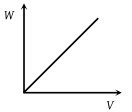(2)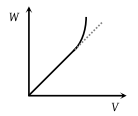(3)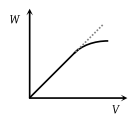(4)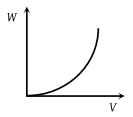Concept Questions :-

Work-Energy theorem
High Yielding Test Series + Question Bank - NEET 2020

Difficulty Level:

Which of the following graphs is correct between kinetic energy (E), potential energy (U) and height (h) from the ground of the particle

(1)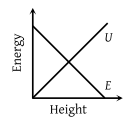(2)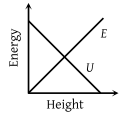(3)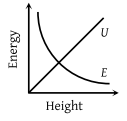(4)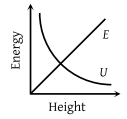Concept Questions :-

Gravitational Potential energy
High Yielding Test Series + Question Bank - NEET 2020

Difficulty Level:

The graph between $\sqrt{E}$ and $\frac{1}{p}$ is (E = kinetic energy and p = momentum)

1.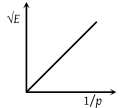2.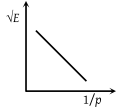3.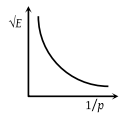4.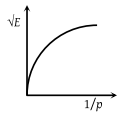Concept Questions :-

Concept of work
High Yielding Test Series + Question Bank - NEET 2020

Difficulty Level:

The force acting on a body moving along x-axis varies with the position of the particle as shown in the fig.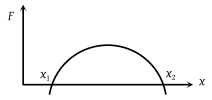The body is in stable equilibrium at

(1) x = x1

(2) x = x2

(3) both x1 and x2

(4) neither x1 nor x2

Concept Questions :-

Potential energy: Relation with force
High Yielding Test Series + Question Bank - NEET 2020

Difficulty Level:

The potential energy of a particle varies with distance x as shown in the graph. The force acting on the particle is zero at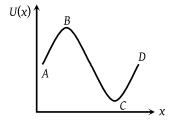(1) C

(2) B

(3) B and C

(4) A and D

Concept Questions :-

Potential energy: Relation with force
High Yielding Test Series + Question Bank - NEET 2020

Difficulty Level:

Figure shows the F-x graph. Where F is the force applied and x is the distance covered by the body along a straight line path. Given that F is in newton and x in metre, what is the work done ?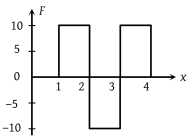(1) 10 J

(2) 20 J

(3) 30 J

(4) 40 J

Concept Questions :-

Work done by variable force
High Yielding Test Series + Question Bank - NEET 2020

Difficulty Level:

The force required to stretch a spring varies with the distance as shown in the figure. If the experiment is performed with the above spring of half length, the line OA will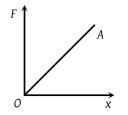(1) Shift towards F-axis

(2) Shift towards X-axis

(3) Remain as it is

(4) Become double in length

Concept Questions :-

Elastic potential energy
High Yielding Test Series + Question Bank - NEET 2020

Difficulty Level:

The graph between E and v is

(1)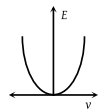(2)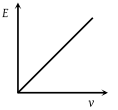(3)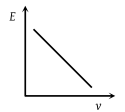(4)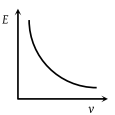Concept Questions :-

Work-Energy theorem
High Yielding Test Series + Question Bank - NEET 2020

Difficulty Level:

A body moves from rest with a constant acceleration. Which one of the following graphs represents the variation of its kinetic energy K with the distance travelled x ?

(1)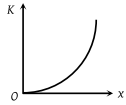(2)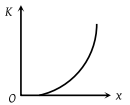(3)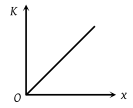(4)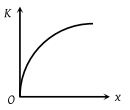High Yielding Test Series + Question Bank - NEET 2020

Difficulty Level:

The diagrams represent the potential energy U of a function of the inter-atomic distance r. Which diagram corresponds to stable molecules found in nature.

(1)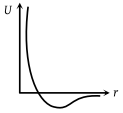(2)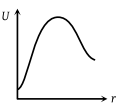(3)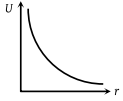(4)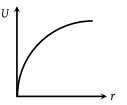Concept Questions :-

Potential energy: Relation with force